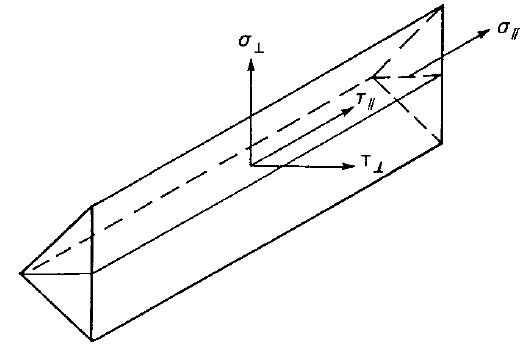# Check of welds according to IS 800

Welds are designed according to IS 800, Cl. 10.5.10.1.1.
$$Butt weldsThe verification of full penetration butt welds is not carried out, as it is assumed to have the same resistance as that of profile, as long as the parent material for the butt weld is superior to that of the profile (IS 800:2007, 10.5.7.1.2).Fillet weldsFillet welds are checked according to IS 800, Cl. 10.5.10.1.1:$f_e = \sqrt{f_a^2 + 3q^2} \le f_{wd} = \frac{f_u}{\sqrt{3} \gamma_{mw}}$where: $$f_e$$ – equivalent stress in weld $$f_a$$ – normal stresses, compression or tension, due to axial force or bending moment $$q$$ – shear stress due to shear force or tension $$f_{wd}$$ – design strength of a fillet weld $$f_u$$ – smaller of the ultimate stress of the weld or of the parent metal; the ultimate strength of the weld electrode is assumed better than of the parent metal $$\gamma_{mw}$$ – partial safety factor for welds – IS 800, Table 5; editable in Code setup The weld diagrams show stress according to the following formula:$\sigma = \sqrt{\sigma_{\perp}^2 + \tau_{\perp}^2 + 3 \tau_{\parallel}^2 }$$$

## Vyzkoušejte program IDEA StatiCa

Stáhněte si zdarma zkušební verzi na 14 dní.